## Definition

### Equivalent definitions in tabular format

No. Shorthand An ideal in a commutative unital ring is termed a radical ideal if ... An ideal$I$ in a commutative unital ring$R$ is termed a radical ideal if ...
1 closed under taking roots whenever a power of an element in the ring lies inside that ideal, the element itself lies inside that ideal For any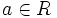$a \in R$ and any positive integer$n$, if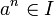$a^n \in I$, then$a \in I$.
2 equals its own radical it equals its own radical in the whole ring$I = \sqrt{I}$ where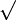$\sqrt{}$ denotes the radical of an ideal, i.e., the set of all elements for which some positive power lies inside the ideal.
3 quotient ring is reduced the quotient ring by the ideal has trivial nilradical (that is, it is a reduced ring) the quotient ring$R/I$ is a reduced ring: whenever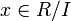$x \in R/I$ and$n$ is a positive integer such that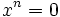$x^n = 0$, then$x = 0$.
4 intersection of prime ideals it can be expressed as an intersection of prime ideals. The intersection is allowed to be finite or infinite. An empty intersection, which would give the whole ring, is also allowed. There exists a collection$P_s, s \in S$ of prime ideals indexed by a set$S$ such that$I = \bigcap_{s \in S} P_s$.$S$ is allowed to be finite or infinite, and is also allowed to be empty.

Note that unlike for the definition of prime ideals, we do not require a radical ideal to be proper, so the whole ring is always a radical ideal in itself.

This article defines a property of an ideal in a commutative unital ring |View other properties of ideals in commutative unital rings
This property of an ideal in a ring is equivalent to the property of the quotient ring being a/an: reduced ring | View other quotient-determined properties of ideals in commutative unital rings

## Examples

### Important ring types

Property of commutative unital rings Characterization of radical ideals in such a ring Examples
principal ideal domain (i.e., an integral domain in which every ideal is a principal ideal) either the zero ideal or a principal ideal generated by a square-free element, i.e., the generating element should be a product of distinct primes In the ring of integers, the radical ideals are precisely the zero ideal and the ideals generated by square-free numbers. Thus, for instance,$6\mathbb{Z}$ and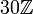$30\mathbb{Z}$ are radical ideals in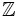$\mathbb{Z}$ but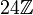$24\mathbb{Z}$ is not.
In the (univariate) polynomial ring over a field, the radical ideals are precisely the zero ideal and the ideals generated by square-free polynomials, which can be described as polynomials with no repeated roots over the algebraic closure, i.e., polynomials that are relatively prime to their derivative polynomial. Thus, for instance,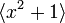$\langle x^2 + 1 \rangle$ and$x^2 - 1$ are radical ideals in$\mathbb{R}[x]$ but$\langle x^2 \rangle$ is not.
Dedekind domain (roughly speaking, admits unique factorization of ideals into prime ideals) either zero or a product of distinct prime ideals Fill this in later
unique factorization domain (unique factorization of elements into primes) principal ideals generated by square-free elements are some, but not all, of the radical ideals. In the multivariate polynomial ring over a field, ...

## Relation with other properties

### Stronger properties

Property Meaning Proof of implication Proof of strictness (reverse implication failure) Intermediate notions Collapse
Maximal ideal proper ideal not contained in any bigger proper ideal
quotient is a field
(via prime ideal) (via prime ideal)  ?
Prime ideal quotient is an integral domain prime implies radical radical not implies prime  ?
Intersection of maximal ideals intersection of maximal ideals Jacobson ring

## Metaproperties

Metaproperty Satisfied? Proof Statement with symbols
intersection-closed property of ideals in commutative unital rings Yes intersection of radical ideals is radical Suppose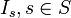$I_s, s \in S$ is a (possibly finite, possibly infinite) collection of radical ideals in a commutative unital ring$R$. Then the intersection$\bigcap_{s \in S} I_s$ is also a radical ideal in$R$.
contraction-closed property of ideals in commutative unital rings Yes Fill this in later If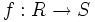$f:R \to S$ is a homomorphism of commutative unital rings, and$I$ is a radical ideal in$S$, then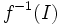$f^{-1}(I)$ is a radical ideal in$R$.
intermediate subring condition for ideals Yes Fill this in later Suppose$I$ is a radical ideal in a commutative unital ring$R$ and$S$ is a unital subring of$R$ that contains$I$. Then,$I$ is also a radical ideal in$S$.
transfer condition for ideals Yes Fill this in later If$I$ is a radical ideal in$R$, and$S$ is a subring of$R$, then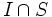$I \cap S$ is a radical ideal in$S$.